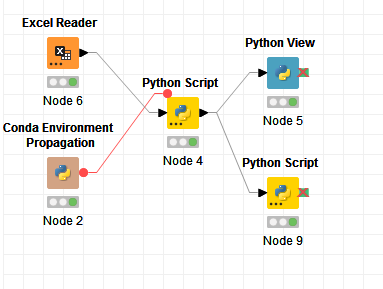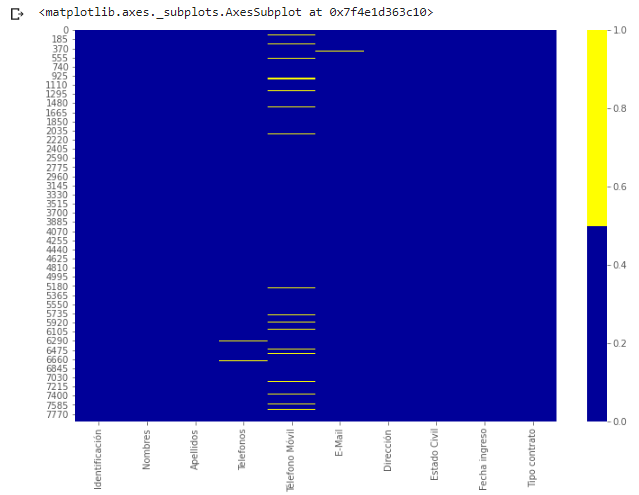# Python view node does not display image output

Hi dear all

i use the python script to generate a heatmap with the packge seaborn, and use the python view node for the output image, but, the node excecute succesfully, However the output of the node shows nothing and there seems to be an error. Attached image of the flow and the code used in the python view node

Code:

"
import pandas as pd
import numpy as np
import seaborn as sns
import matplotlib.pyplot as plt
import matplotlib.mlab as mlab
import matplotlib
plt.style.use(‘ggplot’)
from matplotlib.pyplot import figure
matplotlib.rcParams[‘figure.figsize’] = (12,8)

pd.options.mode.chained_assignment = None
cols = input_table.columns[:10] # first 10 columns
colours = [’#000099’, ‘#ffff00’] # specify the colours - yellow is missing. blue is not missing.
output_image = sns.heatmap(input_table[cols].isnull(), cmap=sns.color_palette(colours))"

Image flow:image in jupyter(spected)Hello @HaroldAndres13 , welcome

Aiming to activate the ‘Image Output’ port in ‘Python View’ node, you have to add the following code to your script from your last line. Renaming the image as ‘image’ will avoid confusion with the output_image :

``````# Create buffer to write into
buffer = BytesIO()

# Create plot and write it into the buffer
image = sns.heatmap(input_table[cols].isnull(), cmap=sns.color_palette(colours))
image.get_figure().savefig(buffer, format='svg')

# The output is the content of the buffer
output_image = buffer.getvalue()
``````

There is a tab in the ‘Python View’ node called ‘Templates’ where you will find a couple of examples more on how to implement the node.

One more example:

Regards

7 Likes

Hi @HaroldAndres13
As you liked the post and if you found it useful. Can you please mark the post as ‘Solution’, so others can easily find the solution for similar challenge?

2 Likes

This topic was automatically closed 7 days after the last reply. New replies are no longer allowed.